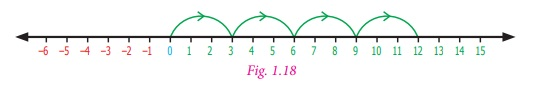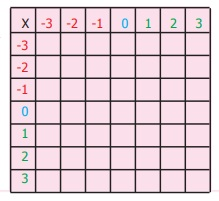Home | | Maths 7th Std | Multiplication of Integers

# Multiplication of Integers

We can draw a number line and visualise the multiplication of integers as repeated addition.

Multiplication of Integers

Situation 1

Ramani and Ravi are playing with pebbles in a heap. Ramani is adding some pebbles to the heap while Ravi removes some pebbles. First, Ramani adds 3 pebbles. Then she adds 3 more pebbles. She repeats this 2 more times. Can you say how many pebbles she added in all? Since adding pebbles is positive we can write ( +3) + ( +3) + ( +3) + ( +3) = +12 or 4 × (+3) = 12

The total number of pebbles added is 12.

Ravi takes out 5 pebbles three times. If we represent “taking out” as a negative integer then ( −5) + ( −5) + ( −5) = −15 or we can simply put it as ( −5) × 3 = −15. Ravi has removed 15 pebbles from the heap. This illustrates that multiplication of negative integers is also repeated addition just like positive integers or whole numbers.

We can draw a number line and visualise the above multiplication of integers as repeated addition.

4 × 3 = 12 (3 is added four times).(−5) × 3 = −15 ((–5) is added three times).We have no difficulty to understand that a positive integer like (+7) multiplied by another positive integer like (+8) is positive (+56). Also, a positive integer like +7 multiplied by a negative integer like –5 is –35 and +5 × −7 = −35 . But what about a negative integer ( −3) multiplied with another negative integer −5 ? To understand this, let us observe the following pattern.

( −5)×3 = −15

( −5)×2 = −10

(−5)×1 = −5

( −5)×0 = 0

( −5)×(−1) = +5

(−5)× (−2) = +10

( −5)× (−3) = 15

Note that how in each step the number increase by 5 from −15 to −10 , from −10 to –5, from –5 to 0. Therefore, the next number in the pattern will be +5 only and not –5. Similarly ( −5) × ( −2) is a positive integer 10. So, the product of two negative integers is always a positive integer.

Try these

1. Find the product of the following

(i) ( −20) × ( −45) = 900

(ii) ( −9) × ( −8) = 72

(iii) ( −30) × 40 × ( −1) = 0

(iv) ( +50) × 2 × ( −10) = –1000

(v) (–30) × 40 × (–1) = 1200

2. Complete the following table by multiplying the integers in the corresponding row and column headers.3. Which of the following is incorrect?

(i) −55×−22×−33<0

Answer: –55 × –22 × –33 = – (55 × 22 × 33) < 0 it is correct

(ii) −1521× 2511 < 0

Answer: –1521 × 2511 < 0 it is correct

(iii) 2512 −1252 < 0

2512 × 1252= 1260 > 0

So 2512 – 1252 < 0 is Incorrect

(iv) +1981× +2000 < 0

+ 1981 × + 2000 = +(1981 × 2000) > 0

So + 1981 × + 2000 < 0 is Incorrect

Think

Can you express the product 15 × 16 as sum or difference of integers?

Yes, here are 4 ways to compute 15 × 16 = +240 .

(i) 15×16 = (10+5)×(10+6) =100+60+50+30 = 240

(ii) 15 ×16 = (20 − 5)× (10 + 6) = 200 +120 + (−50)+ (−20) = 240

(iii) 15 ×16 = (10 + 5)× (20 − 4) = 200 + (−40)+100 + (−20) = 240

(iv) 15 ×16 = (20 − 5)× (20 − 4) = 400 + (−80)+ (−100)+ 20 = 240

From the above pattern one can determine the product of two positive integers, two negative integers, one positive integer with one negative integer.

Example 1.17

Find the value of :

(i) (–35) × (–11)

(ii) 96 × (–20)

(iii) (–5) × 12

(iv) 15×5

(v) 999 x 0

Solution

(i) (–35) × (–11) = 385

(ii) 96 × (–20) = – 1920

(iii) (–5) × 12 = –60

(iv) 15 × 5 = 75

(v) 999 x 0 = 0

Example 1.18

A fruit seller sold 5kg of mangoes at a profit of ₹ 15 per kg and 3kg of apples at a loss of ₹ 30 per kg. Find whether it is a profit or loss.

Solution

Profit of 1kg mangoes = ₹ 15

Profit of 5kg mangoes = ₹15×5

= ₹ 75

Loss of 1kg apples = ₹ 30

Loss of 3kg apples = 30×3

= ₹ 90

Loss = ₹90−₹75

= ₹ 15

Example 1.19

Browsing rates in an internet centre is ₹ 15 per hour. Nila works on the internet for 2 hours in a day for 5 days in a week. How much does she pay?

Solution

Number of hours spent on an internet for a day = 2 hrs

Therefore, number of hours spent on the internet for 5 days = 5 × 2

= 10 hrs

Cost of browsing per hour = ₹ 15

Cost of browsing for 10 hours = 15×10

Therefore, the amount paid by Nila for 5 days = ₹ 150

Tags : Number System | Term 1 Chapter 1 | 7th Maths , 7th Maths : Term 1 Unit 1 : Number System
Study Material, Lecturing Notes, Assignment, Reference, Wiki description explanation, brief detail
7th Maths : Term 1 Unit 1 : Number System : Multiplication of Integers | Number System | Term 1 Chapter 1 | 7th Maths